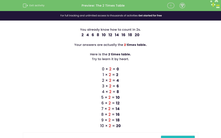# Know the 2 Times Table

In this worksheet, students will answer questions on the 2 times table. Counting in 2s may help.Key stage:  KS 1

Curriculum topic:   Number: Multiplication and Division

Curriculum subtopic:   Solve Multiplication/Division Problems

Popular topics:   Times Table worksheets

Difficulty level:#### Worksheet Overview

You already know how to count in 2s.

2   4   6   8   10   12   14   16   18   20

Here is the 2 times table.

Try to learn it by heart.

0 × 2 = 0

1 × 2 = 2

2 × 2 = 4

3 × 2 = 6

4 × 2 = 8

5 × 2 = 10

6 × 2 = 12

7 × 2 = 14

8 × 2 = 16

9 × 2 = 18

10 × 2 = 20

We're going to practise using it in this activity.

How do you feel about that?### What is EdPlace?

We're your National Curriculum aligned online education content provider helping each child succeed in English, maths and science from year 1 to GCSE. With an EdPlace account you’ll be able to track and measure progress, helping each child achieve their best. We build confidence and attainment by personalising each child’s learning at a level that suits them.

Get started